# Decimate (continuous) VI

## LabVIEW 2018 Help

Edition Date: March 2018
Part Number: 371361R-01
View Product InfoLabVIEW 2016 HelpLabVIEW 2017 HelpLabVIEW 2018 HelpLabVIEW 2019 HelpLabVIEW 2020 Help

Owning Palette: Signal Operation VIs

Requires: Full Development System

Continuously decimates the input sequence X by the decimating factor and the averaging Boolean control. Wire data to the X input to determine the polymorphic instance to use or manually select the instance.

Use the pull-down menu to select an instance of this VI.

 Select an instance Decimate (continuous, DBL)Decimate (continuous, CDB)

## Decimate (continuous, DBL)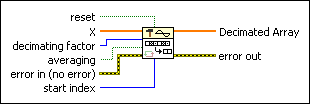reset controls the initialization of the decimation. The default is FALSE. If reset is TRUE or if the VI runs for the first time, LabVIEW initializes the decimation from the sample of X specified by start index. When the VI runs again with reset set to FALSE, LabVIEW initializes the decimation from the final states of the previous call to the VI. To process a large data sequence that consists of smaller blocks, set reset to TRUE for the first block and to FALSE for all remaining blocks. You also can set reset to TRUE at regular intervals of blocks to periodically reset the sample from which the decimation begins.X is the first input sequence.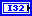decimating factor is the factor by which the VI decimates input sequence X. decimating factor must be greater than zero. The default is 1. If decimating factor is greater than the number of elements in X or less than or equal to zero, this VI sets Decimated Array to an empty array and returns an error.averaging specifies how the VI handles the data points in X. If averaging is FALSE (default), this VI keeps every decimating factor point from X. If averaging is TRUE, each output point in Decimated Array is the mean of the decimating factor input points.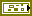error in describes error conditions that occur before this node runs. This input provides standard error in functionality.start index determines from which sample in X the decimation starts if LabVIEW calls the VI for the first time or reset is TRUE. start index must be greater than or equal to zero. The default is 0.Decimated Array returns the decimated sequence of X.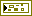error out contains error information. This output provides standard error out functionality.

## Decimate (continuous, CDB)reset controls the initialization of the decimation. The default is FALSE. If reset is TRUE or if the VI runs for the first time, LabVIEW initializes the decimation from the sample of X specified by start index. When the VI runs again with reset set to FALSE, LabVIEW initializes the decimation from the final states of the previous call to the VI. To process a large data sequence that consists of smaller blocks, set reset to TRUE for the first block and to FALSE for all remaining blocks. You also can set reset to TRUE at regular intervals of blocks to periodically reset the sample from which the decimation begins.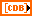X is the complex input sequence for decimation. The number of elements in X must be greater than or equal to the decimating factor.decimating factor is the factor by which the VI decimates input sequence X. decimating factor must be greater than zero. The default is 1. If decimating factor is greater than the number of elements in X or less than or equal to zero, this VI sets Decimated Array to an empty array and returns an error.averaging specifies how the VI handles the data points in X. If averaging is FALSE (default), this VI keeps every decimating factor point from X. If averaging is TRUE, each output point in Decimated Array is the mean of the decimating factor input points.error in describes error conditions that occur before this node runs. This input provides standard error in functionality.start index determines from which sample in X the decimation starts if LabVIEW calls the VI for the first time or reset is TRUE. start index must be greater than or equal to zero. The default is 0.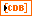Decimated Array returns the decimated sequence of X.error out contains error information. This output provides standard error out functionality.

## Decimate (continuous) Details

If Y represents the output sequence Decimated Array, the Decimate (continuous) VI obtains the elements of the sequence Y using the following equation.

If averaging is FALSE:

Yi=xi*m+s

for i = 0, 1, 2,…, size–1,If averaging is TRUE: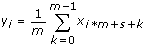for i = 0, 1, 2,…, size–1,where n is the number of elements in X, m is the decimating factor, s is the start index, size is the number of elements in the output sequence Decimated Array,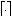gives the smallest integer greater than or equal to the number, and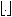gives the largest integer less than or equal to the number.

## Example

Refer to the Continuous Decimating VI in the labview\examples\Signal Processing\Signal Operation directory for an example of using the Decimate (continuous) VI.